CSE 5311 Section 501 Fall 1998
Homework 7
Due: May 6, 1998, in class

1. Compute the product of the polynomials A(x) =and B(x) =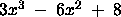using themethod described in class.
2. In the on-line convex-hull problem, we are given the set Q of n points, one point at a time. After receiving each point, we are to compute the convex hull of the points seen so far. Obviously, we could run Graham's scan once for each point, with a total running time of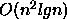. Show how to solve the on-line convex-hull problem in a total of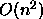time.
3. Consider the bin-packing problem in which we are given n objects having sizes, and we want to pack the objects into a minimum number of unit-sized bins, each holding a total size not exceeding 1 (this is Problem 37-1 in the textbook).

a.
Prove that the problem of determining the minimum number of bins required is NP-hard. (Hint: reduce from the subset-sum problem).

b.
Argue that the optimal number of bins required is at least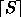.

4. Consider the subgraph isomorphism problem (SI) that takes two graphsand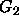and asks whetheris a subgraph of. Prove that SI is NP-Complete by reducing from the clique problem.

SI: Given two graphs, G = (V1, E1) and H = (v2, e2), there is a subgraph of G that is isomorphic to H; that is, a subset V <= V1 and a subset E <= E1 such that |V| = |V2|, |e| = |E2|, and there exists a one-to-one function f: V2 -> V satisfying u, v in E2 if and only if {f(u), f(v) in E.

5. Use the Boyer-Moore-Matching algorithm to implement a grep utility. Grep takes as input a string s and a file f, and returns each line of f that includes an instance of the string s. Send all code, sample files, and compile information to cs5311-turnin@cse.